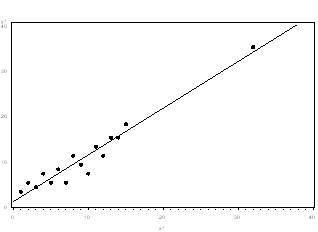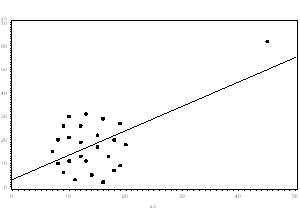# transformation

### What Is Specification Error in Statistical Models?

June 8th, 2022 by

When we think about model assumptions, we tend to focus on assumptions like independence, normality, and constant variance. The other big assumption, which is harder to see or test, is that there is no specification error. The assumption of linearity is part of this, but it’s actually a bigger assumption.

What is this assumption of no specification error? (more…)

### Member Training: Using Transformations to Improve Your Linear Regression Model

March 5th, 2018 by

Transformations don’t always help, but when they do, they can improve your linear regression model in several ways simultaneously.They can help you better meet the linear regression assumptions of normality and homoscedascity (i.e., equal variances). They also can help avoid some of the artifacts caused by boundary limits in your dependent variable — and sometimes even remove a difficult-to-interpret interaction.

### Member Training: Transformations & Nonlinear Effects in Linear Models

May 7th, 2015 by

Why is it we can model non-linear effects in linear regression?What the heck does it mean for a model to be “linear in the parameters?” (more…)

### Outliers: To Drop or Not to Drop

September 17th, 2008 by

Should you drop outliers? Outliers are one of those statistical issues that everyone knows about, but most people aren’t sure how to deal with.  Most parametric statistics, like means, standard deviations, and correlations, and every statistic based on these, are highly sensitive to outliers.

And since the assumptions of common statistical procedures, like linear regression and ANOVA, are also based on these statistics, outliers can really mess up your analysis.

Despite all this, as much as you’d like to, it is NOT acceptable to drop an observation just because it is an outlier.  They can be legitimate observations and are sometimes the most interesting ones.  It’s important to investigate the nature of the outlier before deciding.

1. If it is obvious that the outlier is due to incorrectly entered or measured data, you should drop the outlier:

For example, I once analyzed a data set in which a woman’s weight was recorded as 19 lbs.  I knew that was physically impossible.  Her true weight was probably 91, 119, or 190 lbs, but since I didn’t know which one, I dropped the outlier.

This also applies to a situation in which you know the datum did not accurately measure what you intended.  For example, if you are testing people’s reaction times to an event, but you saw that the participant is not paying attention and randomly hitting the response key, you know it is not an accurate measurement.

2. If the outlier does not change the results but does affect assumptions, you may drop the outlier.  But note that in a footnote of your paper.

Neither the presence nor absence of the outlier in the graph below would change the regression line:3. More commonly, the outlier affects both results and assumptions.  In this situation, it is not legitimate to simply drop the outlier.  You may run the analysis both with and without it, but you should state in at least a footnote the dropping of any such data points and how the results changed.4. If the outlier creates a strong association, you should drop the outlier and should not report any association from your analysis.

In the following graph, the relationship between X and Y is clearly created by the outlier.  Without it, there is no relationship between X and Y, so the regression coefficient does not truly describe the effect of X on Y.So in those cases where you shouldn’t drop the outlier, what do you do?

One option is to try a transformation.  Square root and log transformations both pull in high numbers.  This can make assumptions work better if the outlier is a dependent variable and can reduce the impact of a single point if the outlier is an independent variable.

Another option is to try a different model.  This should be done with caution, but it may be that a non-linear model fits better.  For example, in example 3, perhaps an exponential curve fits the data with the outlier intact.

Whichever approach you take, you need to know your data and your research area well.  Try different approaches, and see which make theoretical sense.